## Sum top N values or top N values with criteria in Excel

This step-by-step tutorial demonstrates how to use the SUMPRODUCT function with the LARGE function to sum the top N values in a range and sum the top N values in a range with criteria in Excel.

How to get the sum of top n values in Excel?
How to get the sum of top n values with criteria in Excel?

#### How to get the sum of top n values in Excel?

As shown in the screenshot below, to sum the top 3 values and the top 6 values in range B5:B15, you can do the following steps.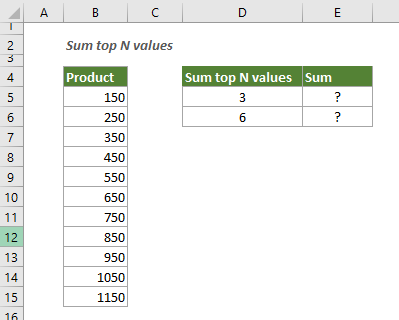Generic Formulas

=SUMPRODUCT(LARGE(data_range,{1,2,...,N}))

Arguments

Data_range: The range from which you want to sum the top N values;
{1,2,...,N}: Represents the top values you want to sum. Here the number 1 represents the first top; the number 2 represents the second top; the N represents the Nth top. If you want to sum top 3 values, then N is 3.

How to use this formula?

Select a cell, copy or enter the formula below and press the Enter key to get the sum of top 3 values.

=SUMPRODUCT(LARGE(B5:B15,{1,2,3}))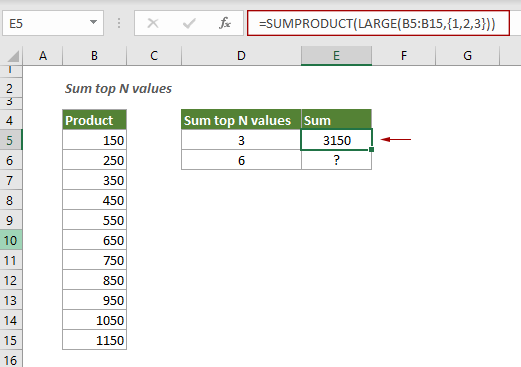To get the sum of top 6 values, apply the formula below.

=SUMPRODUCT(LARGE(B5:B15,{1,2,3,4,5,6}))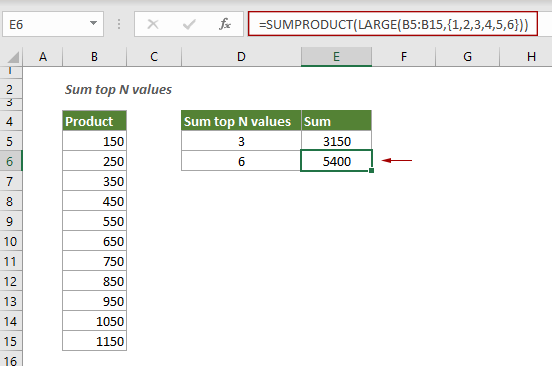Note: To sum any top n values from a range, please change the {1,2,...N} according to your needs.

Explanation of the formula

=SUMPRODUCT(LARGE(B5:B15,{1,2,3}))

LARGE(B5:B15,{1,2,3}): The LARGE function returns an array of result with the 1st, 2nd and 3rd top value in range B5:B15: {1150,1050,950};
SUMPRODUCT({1150,1050,950}): Then the SUMPRODUCT function adds up all numbers in the array and returns the final result as 3150.

#### How to get the sum of top n values with criteria in Excel?

The SUMPRODUCT function with the LARGE function can also be applied to get the sum of top n values with criteria in Excel.

Look at the following example, to sum the top 3 amounts of KTE, you can do as follows.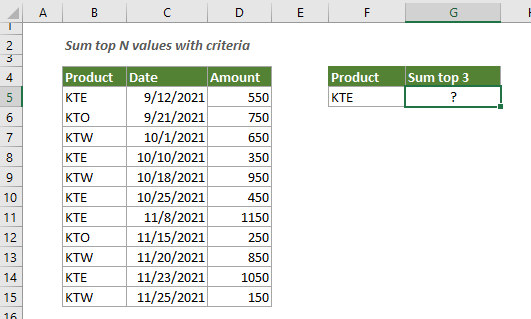Generic formula

=SUMPRODUCT(LARGE(data_range,{1,2,...,N}))

Arguments

Data_range: The range from which you want to sum the top N values;
{1,2,...,N}: Represents the top values you want to sum. Here the number 1 represents the first top; the number 2 represents the second top; the N represents the Nth top. If you want to sum top 3 values, then N is 3.

How to use this formula?

Select a cell, copy or enter the formula below and press the Enter key to get the result.

=SUMPRODUCT(LARGE((B5:B15=F5)*(D5:D15),{1,2,3}))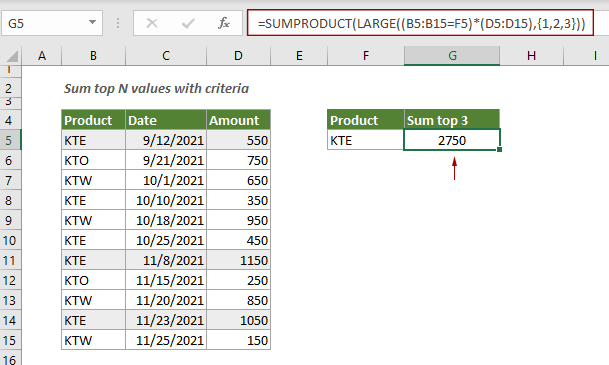Note: To sum any top n values, please change the {1,2,...N} according to your needs.

Explanation of this formula

=SUMPRODUCT(LARGE((B5:B15=F5)*(D5:D15),{1,2,3}))

LARGE((B5:B15=F5)*(D5:D15),{1,2,3})
(B5:B15=F5): Here checks if each cell in B5:B15 equals to “KTE” pulled from F5, and returns an array {TRUE;FALSE;FALSE;TRUE;FALSE;TRUE;TRUE;FALSE;FALSE;TRUE;FALSE};
{TRUE;FALSE;FALSE;TRUE;FALSE;TRUE;TRUE;FALSE;FALSE;TRUE;FALSE}*(D5:D15): The TRUEs and FALSEs in the array are converted to 1s and 0s, then multiplied by the values in D5:D15. You will get a new array like this {550;0;0;350;0;450;1150;0;0;1050;0};
LARGE({550;0;0;350;0;450;1150;0;0;1050;0},{1,2,3}): The LARGE function returns the top 3 values in the array: {1150,1050,550}.
SUMPRODUCT({1150,1050,550}): The SUMPRODUCT function adds up all values in the array and returns the result as 2750.

#### Related functions

Excel SUMPRODUCT function
The Excel SUMPRODUCT function can be used to multiply two or more columns or arrays together, and then get the sum of products.

Excel LARGE function
The Excel LARGE function returns the k-th largest value in a data set.

#### Related formulas

Sum multiple columns if one criterion is met
This tutorial will guide you how to sum values in multiple columns based on the same criterion in Excel.

Sum the last N columns
To sum values in the last n columns, normally you may need to calculate the sum of each column and then add up the results to get the final sum. This tutorial provides a formula based on the INDEX function to help you easily get it done.

Sum values based on column or both column and row
This tutorial provides different formulas based on the SUMPRODUCT function to help you sum values based on a column header, and sum values based on both column and row criteria.

Sum values in last N days based on criteria
To sum values in last N days based on specific criteria in Excel, you can apply a formula based on the SUMIF and TODAY function.

### The Best Office Productivity Tools

#### Kutools for Excel - Helps You To Stand Out From Crowd

 Popular Features: Find, Highlight or Identify Duplicates  |  Delete Blank Rows  |  Combine Columns or Cells without Losing Data  |  Round without Formula ... Super VLookup: Multiple Criteria  |  Multiple Value  |  Across Multi-Sheets  |  Fuzzy Lookup... Adv. Drop-down List: Easy Drop Down List  |  Dependent Drop Down List  |  Multi-select Drop Down List... Column Manager: Add a Specific Number of Columns  |  Move Columns  |  Toggle Visibility Status of Hidden Columns  |  Compare Columns to Select Same & Different Cells ... Featured Features: Grid Focus  |  Design View  |  Big Formula Bar  |  Workbook & Sheet Manager | Resource Library (Auto Text)  |  Date Picker  |  Combine Worksheets  |  Encrypt/Decrypt Cells  |  Send Emails by List  |  Super Filter  |  Special Filter (filter bold/italic/strikethrough...) ... Top 15 Toolsets:  12 Text Tools (Add Text, Remove Characters ...)  |  50+ Chart Types (Gantt Chart ...)  |  40+ Practical Formulas (Calculate age based on birthday ...)  |  19 Insertion Tools (Insert QR Code, Insert Picture from Path ...)  |  12 Conversion Tools (Numbers to Words, Currency Conversion ...)  |  7 Merge & Split Tools (Advanced Combine Rows, Split Excel Cells ...)  |  ... and more

Kutools for Excel Boasts Over 300 Features, Ensuring That What You Need is Just A Click Away...#### Office Tab - Enable Tabbed Reading and Editing in Microsoft Office (include Excel)

• One second to switch between dozens of open documents!
• Reduce hundreds of mouse clicks for you every day, say goodbye to mouse hand.
• Increases your productivity by 50% when viewing and editing multiple documents.
• Brings Efficient Tabs to Office (include Excel), Just Like Chrome, Edge and Firefox.